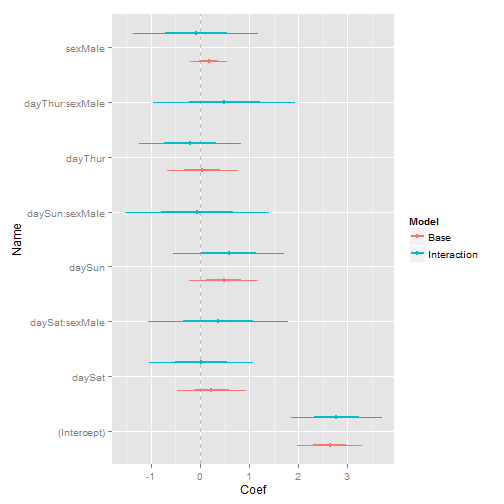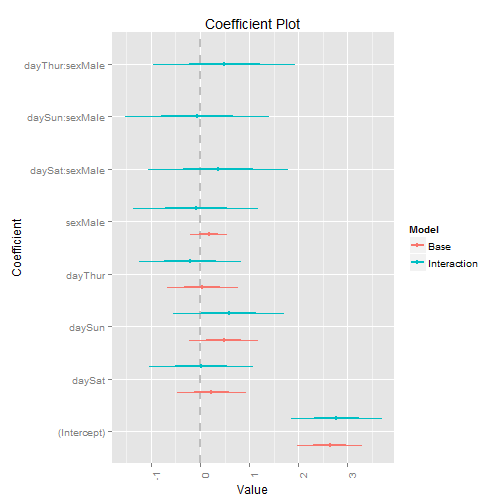An often requested feature for Hadley Wickham's ggplot2 package is the ability to vertically dodge points, lines and bars. There has long been a function to shift geoms to the side when the x-axis is categorical: position_dodge. However, no such function exists for vertical shifts when the y-axis is categorical. Hadley usually responds by saying it should be easy to build, so here is a hacky patch.

All I did was copy the old functions (geom_dodge, collide, pos_dodge and PositionDodge) and make them vertical by swapping y's with x's, height with width and vice versa. It's hacky and not tested but seems to work as I'll show below.

First the new functions:

``````require(proto)
``````
``````## Loading required package: proto
``````
``````collidev <- function(data, height = NULL, name, strategy, check.height = TRUE) {
if (!is.null(height)) {
if (!(all(c("ymin", "ymax") %in% names(data)))) {
data <- within(data, {
ymin <- y - height/2
ymax <- y + height/2
})
}
} else {
if (!(all(c("ymin", "ymax") %in% names(data)))) {
data\$ymin <- data\$y
data\$ymax <- data\$y
}
heights <- unique(with(data, ymax - ymin))
heights <- heights[!is.na(heights)]
if (!zero_range(range(heights))) {
warning(name, " requires constant height: output may be incorrect",
call. = FALSE)
}
height <- heights
}
data <- data[order(data\$ymin), ]
intervals <- as.numeric(t(unique(data[c("ymin", "ymax")])))
intervals <- intervals[!is.na(intervals)]
if (length(unique(intervals)) > 1 & any(diff(scale(intervals)) < -1e-06)) {
warning(name, " requires non-overlapping y intervals", call. = FALSE)
}
if (!is.null(data\$xmax)) {
ddply(data, .(ymin), strategy, height = height)
} else if (!is.null(data\$x)) {
transform(ddply(transform(data, xmax = x), .(ymin), strategy, height = height),
x = xmax)
} else {
stop("Neither x nor xmax defined")
}
}

pos_dodgev <- function(df, height) {
n <- length(unique(df\$group))
if (n == 1)
return(df)
if (!all(c("ymin", "ymax") %in% names(df))) {
df\$ymin <- df\$y
df\$ymax <- df\$y
}
d_width <- max(df\$ymax - df\$ymin)
diff <- height - d_width
groupidx <- match(df\$group, sort(unique(df\$group)))
df\$y <- df\$y + height * ((groupidx - 0.5)/n - 0.5)
df\$ymin <- df\$y - d_width/n/2
df\$ymax <- df\$y + d_width/n/2
df
}

position_dodgev <- function(width = NULL, height = NULL) {
PositionDodgev\$new(width = width, height = height)
}

PositionDodgev <- proto(ggplot2:::Position, {
objname <- "dodgev"

if (empty(data))
return(data.frame())
check_required_aesthetics("y", names(data), "position_dodgev")

collidev(data, .\$height, .\$my_name(), pos_dodgev, check.height = TRUE)
}

})
``````

Now that they are built we can whip up some example data to show them off. Since this was inspired by a refactoring of my coefplot package I will use a deconstructed sample.

``````# get tips data
data(tips, package = "reshape2")

# fit some models
mod1 <- lm(tip ~ day + sex, data = tips)
mod2 <- lm(tip ~ day * sex, data = tips)

# build data/frame with coefficients and confidence intervals and combine
# them into one data.frame
require(coefplot)
``````
``````## Loading required package: coefplot
``````
``````## Loading required package: ggplot2
``````
``````df1 <- coefplot(mod1, plot = FALSE, name = "Base", shorten = FALSE)
df2 <- coefplot(model = mod2, plot = FALSE, name = "Interaction", shorten = FALSE)
theDF <- rbind(df1, df2)
theDF
``````
``````##    LowOuter HighOuter LowInner HighInner     Coef            Name Checkers
## 1    1.9803    3.3065  2.31183    2.9750  2.64340     (Intercept)  Numeric
## 2   -0.4685    0.9325 -0.11822    0.5822  0.23202          daySat      day
## 3   -0.2335    1.1921  0.12291    0.8357  0.47929          daySun      day
## 4   -0.6790    0.7672 -0.31745    0.4056  0.04408         dayThur      day
## 5   -0.2053    0.5524 -0.01589    0.3630  0.17354         sexMale      sex
## 6    1.8592    3.7030  2.32016    3.2421  2.78111     (Intercept)  Numeric
## 7   -1.0391    1.0804 -0.50921    0.5506  0.02067          daySat      day
## 8   -0.5430    1.7152  0.02156    1.1507  0.58611          daySun      day
## 9   -1.2490    0.8380 -0.72725    0.3163 -0.20549         dayThur      day
## 10  -1.3589    1.1827 -0.72349    0.5473 -0.08811         sexMale      sex
## 11  -1.0502    1.7907 -0.34000    1.0804  0.37022  daySat:sexMale  day:sex
## 12  -1.5324    1.4149 -0.79560    0.6781 -0.05877  daySun:sexMale  day:sex
## 13  -0.9594    1.9450 -0.23328    1.2189  0.49282 dayThur:sexMale  day:sex
##          CoefShort       Model
## 1      (Intercept)        Base
## 2           daySat        Base
## 3           daySun        Base
## 4          dayThur        Base
## 5          sexMale        Base
## 6      (Intercept) Interaction
## 7           daySat Interaction
## 8           daySun Interaction
## 9          dayThur Interaction
## 10         sexMale Interaction
## 11  daySat:sexMale Interaction
## 12  daySun:sexMale Interaction
## 13 dayThur:sexMale Interaction
``````
``````# build the plot
require(ggplot2)
require(plyr)
``````
``````## Loading required package: plyr
``````
``````ggplot(theDF, aes(y = Name, x = Coef, color = Model)) + geom_vline(xintercept = 0,
linetype = 2, color = "grey") + geom_errorbarh(aes(xmin = LowOuter, xmax = HighOuter),
height = 0, lwd = 0, position = position_dodgev(height = 1)) + geom_errorbarh(aes(xmin = LowInner,
xmax = HighInner), height = 0, lwd = 1, position = position_dodgev(height = 1)) +
geom_point(position = position_dodgev(height = 1), aes(xmax = Coef))
``````Compare that to the multiplot function in coefplot that was built using geom_dodge and coord_flip.

``````multiplot(mod1, mod2, shorten = F, names = c("Base", "Interaction"))
``````With the exception of the ordering and plot labels, these charts are the same. The main benefit here is that avoiding coord_flip still allows the plot to be faceted, which was not possible with coord_flip.

Hopefully Hadley will be able to take these functions and incorporate them into ggplot2.Jared Lander is the Chief Data Scientist of Lander Analytics a New York data science firm, Adjunct Professor at Columbia University, Organizer of the New York Open Statistical Programming meetup and the New York and Washington DC R Conferences and author of R for Everyone.